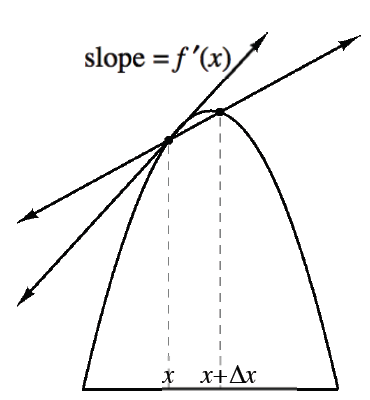### Home > APCALC > Chapter 3 > Lesson 3.1.2 > Problem3-34

3-34.

In many textbooks the derivative (or IROC) is described in terms of $x$ and $Δx$ (delta $x$) instead of $x$ and $h$

1. Explain why $h$ and $Δx$ are equivalent.

$Δx$ can be translated as 'change in $x$.' $h$ typically appears in the expression: $(x + h) −x$. How are these equivalent?

2. Use the diagram at right and the definition of IROC to write the slope of the tangent line in terms of $x$ and $\Delta x$.

'Definition of the Derivative' is the same thing as 'Instantaneous Rate of Change': IROC

$f'(x)=\lim\limits_{h\rightarrow 0}\frac{f(x+h)-f(x)}{(x+h)-h}=\lim\limits_{h\rightarrow 0}\frac{f(x+h)-f(x)}{h}...$